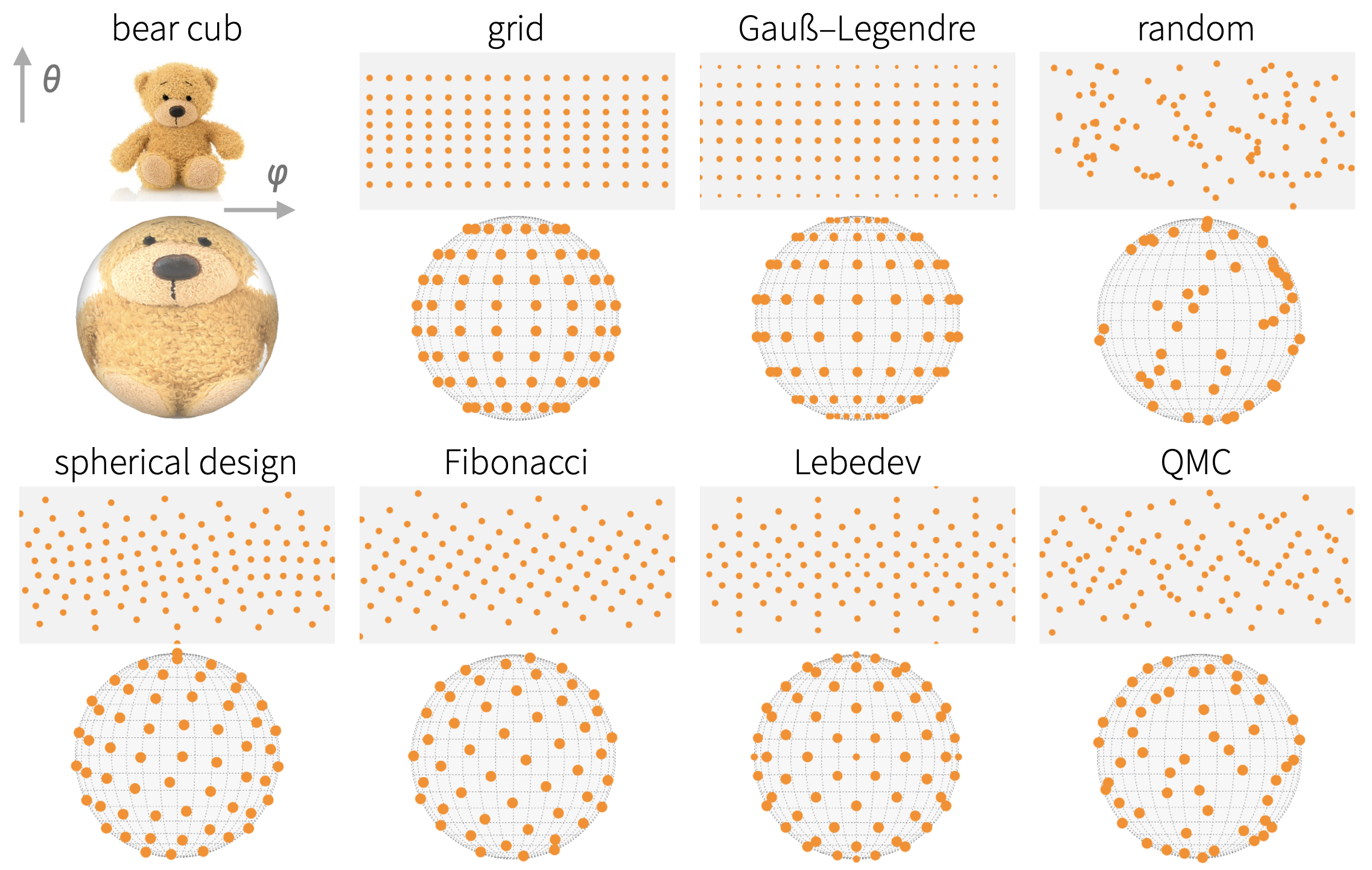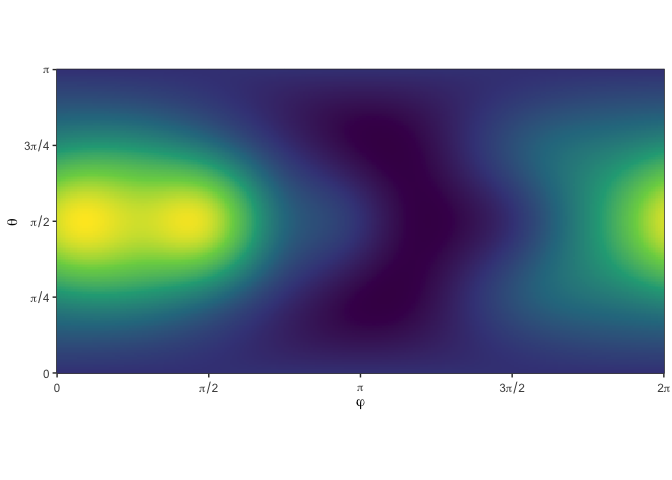cubs is a utility package wrapping several commonly-used spherical cubature rules in a convenient interface:

• Lebedev
• Spherical t-Designs
• Gauß-Legendre
• Fibonacci
• grid
• Quasi Monte-Carlo
• randomcubs

## Installation

You can install from GitHub with:

# install.package('remotes')
remotes::install_github('nano-optics/cubs')

## Simple example

Let’s request a Lebedev cubature with approximately 10 points,

cubs(N = 10, 'lebedev')
phi theta weight
1 0.0000000 1.5707963 0.0666667
2 3.1415927 1.5707963 0.0666667
3 1.5707963 1.5707963 0.0666667
4 -1.5707963 1.5707963 0.0666667
5 1.5707963 0.0000000 0.0666667
6 1.5707963 3.1415927 0.0666667
7 0.7853982 0.9553166 0.0750000
8 0.7853982 2.1862760 0.0750000
9 -0.7853982 0.9553166 0.0750000
10 -0.7853982 2.1862760 0.0750000
11 2.3561945 0.9553166 0.0750000
12 2.3561945 2.1862760 0.0750000
13 -2.3561945 0.9553166 0.0750000
14 -2.3561945 2.1862760 0.0750000

Lebedev, 10 points requested

Let’s try a known integrand,

f1(x, y, z) = 1 + x + y2 + x2y + x4 + y5 + x2y2z2

with the usual spherical coordinates.

We want to estimate the integral $I=\frac{1}{4\pi }{\int }_{0}^{\pi }{\int }_{0}^{2\pi }f\left(\phi ,\theta \right)sin\theta \phantom{\rule{0.167em}{0ex}}\mathrm{\text{d}}\phi \mathrm{\text{d}}\theta$ numerically, i.e. with a spherical cubature

$I\approx \sum _{{\phi }_{i},{\theta }_{i}}^{i=1\dots N}f\left({\phi }_{i},{\theta }_{i}\right){w}_{i}$We compare the exact value, 216π/35, to the Lebedev cubature for increasing number of points.

source value
lebedev N=14 19.5476876223365
lebedev N=26 19.3881146621542
exact 19.3881146621542

## Acknowledgements

This package merely wraps existing rules in a convenient interface; the original cubature points were obtained from:

• Lebedev: from John Burkardt’s webpage, using the SPHERE_LEBEDEV_RULE C routine. The routine itself implements the original reference by Lebedev and Laikov

Vyacheslav Lebedev, Dmitri Laikov, A quadrature formula for the sphere of the 131st algebraic order of accuracy, Russian Academy of Sciences Doklady Mathematics, Volume 59, Number 3, 1999, pages 477-481.

• Spherical t-Designs: from Rob Womersley’s webpage, using the provided tables of Spherical t-designs (SF29-Nov-2012).

• Gauß-Legendre: we use the gauss.quad routine from the statmod package to compute 1D quadrature nodes on [ − 1, 1], and take a cartesian product with a mid-point rule along φ.

• QMC: we use the halton routine from the randtoolbox package to generate a 2D low-discrepancy sequence of points in [0, 1] × [0, 1].

Fibonacci, grid, and random are implemented directly in the package.